# Thomas' Functional Interpreter

Thomas Uhrig · July 27, 2012

I just finished a small functional interpreter for an university course. To be precise, the interpreter itself is not functional, but it understands a functional, LISP-like syntax. The whole project is written in Java 7 and provides an interface to all Java classes on the class-path, too.

I also tried to create a small IDE in Swing. I built an editor with syntax-highlighting and code-completion (thanks to the RSyntaxTextArea project), an environment inspector and a small debugger to keep track of the stack. Take a look at it.

I called it ThoFu for Thomas’ Functional Interpreter. You can get some brief impressions from the images below.

The whole project is open source, you can find it on my GitHub account. It contains the actual code, all libraries, screen-shots, unit tests and (of course) some documentation like UML diagrams.

## Screenshots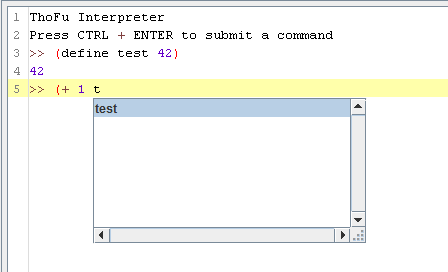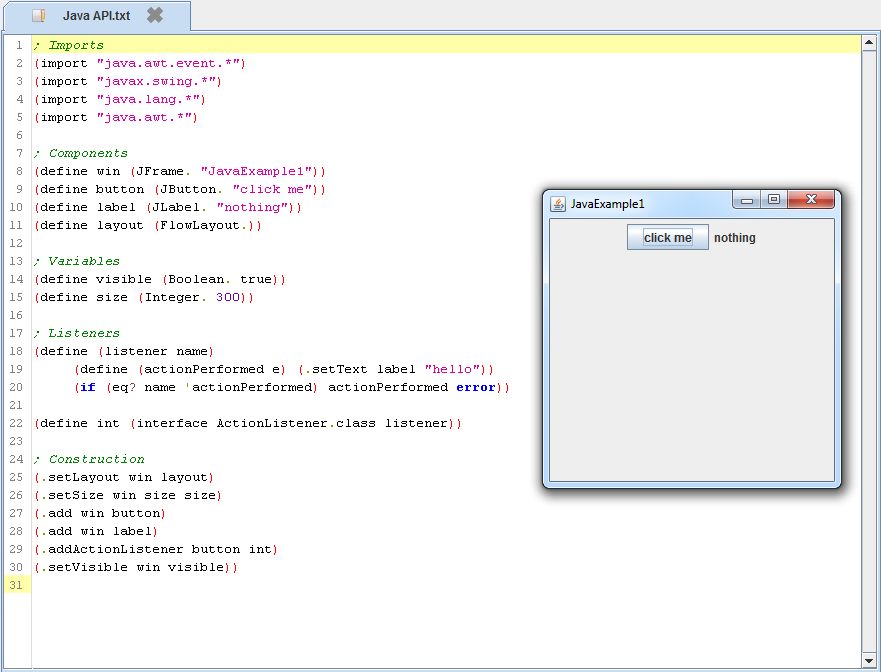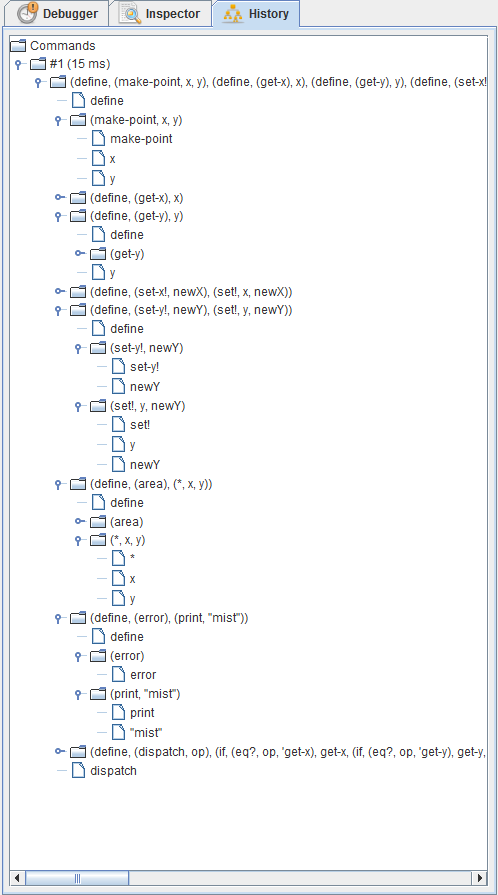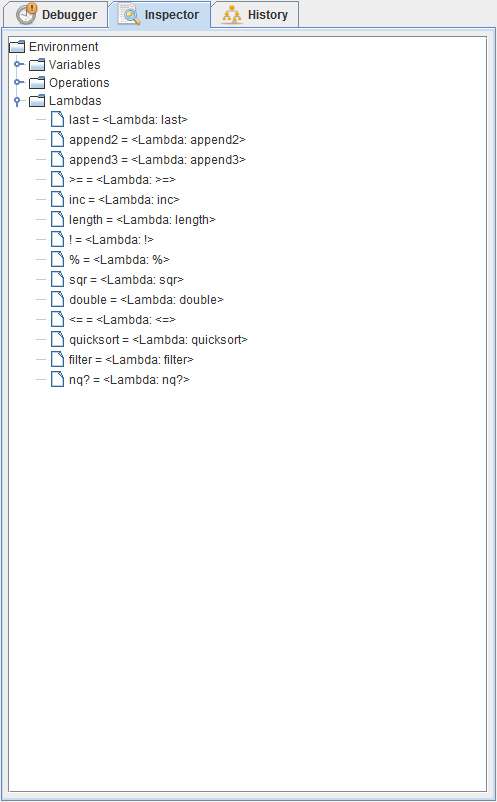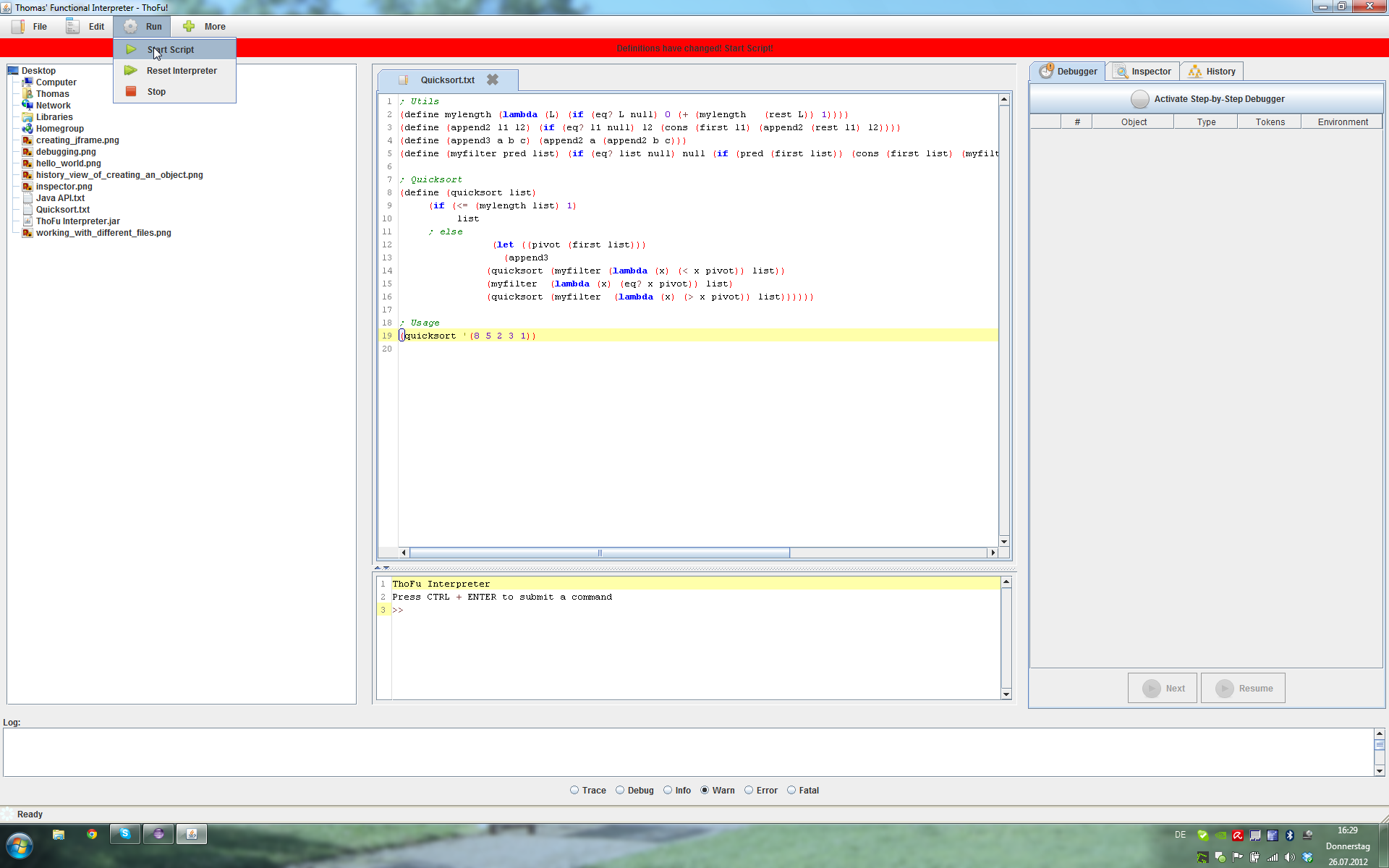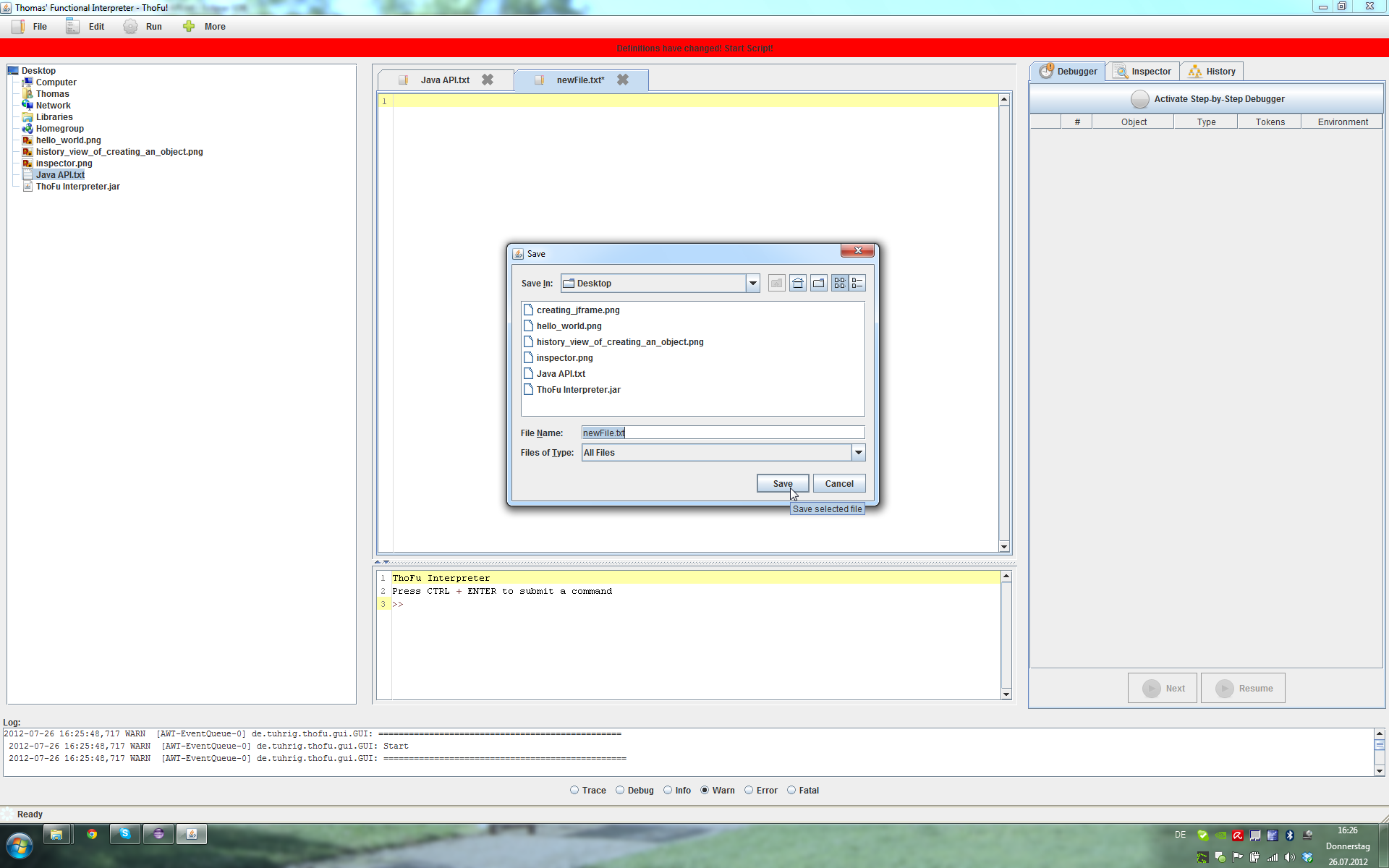## Examples

And this is how it goes. First a simple “hello world” program:

``````(print "hello world")
``````

Now, a more sophisticated one. Here’s a quicksort-algorithm for my interpreter. It contains some user-defined functions, multi-line statements and some comments.

``````; Utils
(define mylength (lambda (L) (if (eq? L null) 0 (+ (mylength   (rest L)) 1))))
(define (append2 l1 l2) (if (eq? l1 null) l2 (cons (first l1) (append2 (rest l1) l2))))
(define (append3 a b c) (append2 a (append2 b c)))
(define (myfilter pred list) (if (eq? list null) null (if (pred (first list)) (cons (first list) (myfilter pred (rest list))) (myfilter pred (rest list)))))

; Quicksort
(define (quicksort list)
(if (<= (mylength list) 1)
list
; else
(let ((pivot (first list)))
(append3
(quicksort (myfilter (lambda (x) (< x pivot)) list))
(myfilter  (lambda (x) (eq? x pivot)) list)
(quicksort (myfilter  (lambda (x) (> x pivot)) list))))))

; Usage
(quicksort '(8 5 2 3 1))
``````

And this is how the Java API works. The code will create a `JFrame` with a `JButton` and a `JLabel`. When you press the button, the listener will change the text of the label from “click me” to “hello”. The syntax is inspired by the Java Dot Notation by Peter Norvig. I used the Apache Commons Lang library for the reflection-tricks to load class and so on.

``````; Imports
(import "java.awt.event.\*")
(import "javax.swing.\*")
(import "java.lang.\*")
(import "java.awt.\*")

; Components
(define win (JFrame. "JavaExample1"))
(define button (JButton. "click me"))
(define label (JLabel. "nothing"))
(define layout (FlowLayout.))

; Variables
(define visible (Boolean. true))
(define size (Integer. 300))

; Listeners
(define (listener name)
(define (actionPerformed e) (.setText label "hello"))
(if (eq? name 'actionPerformed) actionPerformed error))

(define int (interface ActionListener.class listener))

; Construction
(.setLayout win layout)
(.setSize win size size)